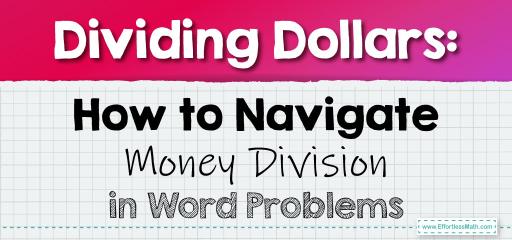# Dividing Dollars: How to Navigate Money Division in Word Problems

Money division problems often arise in scenarios like splitting bills, sharing costs, or determining unit prices. Understanding how to tackle these problems is crucial for making informed financial decisions. Let's explore some word problems that involve dividing money amounts.## Dividing Money Quantities: Word Problems

### Example 1:

John and his three friends want to equally split the cost of a $48 meal. How much does each person pay? Solution Process: Divide the total cost by the number of people.$48 ÷ 4 = $12 Answer: Each person pays$12.

The Absolute Best Book for 5th Grade Students

### Example 2:

A box of 6 chocolates costs $18. What is the cost of a single chocolate? Solution Process: Divide the total cost by the number of chocolates.$18 ÷ 6 = $3 Answer: Each chocolate costs$3.

Dividing money quantities into word problems helps us understand the distribution of costs and the value of individual items. By systematically dividing the total amount by the number of units or people, you can easily determine individual shares or unit prices. Whether you’re splitting a bill, determining the cost of a single item, or budgeting for an event, these division skills are invaluable. So, the next time you’re faced with a division-related money problem, approach it with clarity and confidence, knowing you’re well-equipped to find the solution!

### Practice Questions:

1. Four friends went to a concert with tickets costing a total of $200. How much did each ticket cost? 2. A pack of 10 pencils costs$5. What is the cost of a single pencil?

3. Sarah bought 5 identical shirts for a total of $125. How much does each shirt cost? 4. A$36 cake is to be equally shared among 8 people in terms of cost. How much does each person contribute?

A Perfect Book for Grade 5 Math Word Problems!

1. $50 2.$0.50

3. $25 4.$4.50

The Best Math Books for Elementary Students

### What people say about "Dividing Dollars: How to Navigate Money Division in Word Problems - Effortless Math: We Help Students Learn to LOVE Mathematics"?

No one replied yet.

X
51% OFF

Limited time only!

Save Over 51%

SAVE $15 It was$29.99 now it is \$14.99